# Parametric and non-parametric statistics on event-related fields

## Introduction

The goal of this tutorial is to provide a gentle introduction into the different options that are implemented for statistical analysis. Here we will use event-related fields (ERFs), because they are more familiar to most of the audience and easier to visualize. We will show how to do basic statistical testing using the MATLAB statistics toolbox and compare the results with that from using the ft_timelockstatistics function. Topics that will be covered are parametric statistics on a single channel and time-window, the multiple comparison problem (MCP), non-parametric randomization testing and cluster-based testing.

This tutorial uses the same MEG language dataset as some of the other tutorials where analyses were done on the single subject level. However, here we will deal with (statistical) analyses on the group level. We will look at how to test statistical differences among conditions in a within-subjects design. The ERF dataset in this tutorial contains data from all 10 subjects that participated in the experiment. The ERF data was obtained using ft_timelockanalysis. For the purpose of inspecting your data visually, we also use ft_timelockgrandaverage to calculate the grand average across participants, which can be used for subsequent visualization.

You can download the ERF_orig.mat data structure with the average for each subject.

The tutorial assumes that the preprocessing and averaging steps are already clear for the reader. If this is not the case, you can read about those steps in other tutorials.

Note that in this tutorial we will not provide detailed information about statistics on channel-level power spectra, time-frequency representations of power (as obtained from ft_freqanalysis), nor on source-level statistics. However, FieldTrip does have similar statistical options for frequency data: at the channel-level we have the ft_freqstatistics function, and on the source-level (statistics on source reconstructed activity), we have the ft_sourcestatistics function, the latter works on data obtained from ft_sourceanalysis).

A more thorough explanation of randomization tests and cluster-based statistics can be found in the Cluster-based permutation tests on event-related fields and the Cluster-based permutation tests on time-frequency data tutorials.

## Background

The topic of this tutorial is the statistical analysis of MEG and EEG data. In experiments, the data is usually collected in different experimental conditions, and the experimenter wants to know, by means of statistical testing, whether there is a difference in the data observed in these conditions. In statistics, a result (for example, a difference among conditions) is statistically significant if it is unlikely to have occurred by chance according to a predetermined threshold probability, the significance level.

An important feature of the MEG and EEG data is that it has a spatial temporal structure, i.e. the data is sampled at multiple time-points and channels. The nature of the data influences which kind of statistics is the most suitable for comparing conditions. If the experimenter is interested in a difference in the signal at a certain time-point and channel, then the more widely used parametric tests are also sufficient. If it is not possible to predict where the differences are, then many statistical comparisons are necessary which lead to the multiple comparisons problem (MCP). The MCP arises from the fact that the effect of interest (i.e., a difference between experimental conditions) is evaluated at an extremely large number of (channel,time)-pairs. This number is usually in the order of several thousands. Now, the MCP involves that, due to the large number of statistical comparisons (one per (channel,time)-pair), it is not possible to control the so called family-wise error rate (FWER) by means of the standard statistical procedures that operate at the level of single (channel,time)-pairs. The FWER is the probability, under the hypothesis of no effect, of falsely concluding that there is a difference between the experimental conditions at one or more (channel,time)-pairs. A solution of the MCP requires a procedure that controls the FWER at some critical alpha-level (typically, 0.05 or 0.01). The FWER is also called the false alarm rate.

When parametric statistics are used, one method that addresses this problem is the so-called Bonferroni correction. The idea is if the experimenter is conducting n number of statistical tests then each of the individual tests should be tested under a significance level that is divided by n. The Bonferroni correction was derived from the observation that if n tests are performed with an alpha significance level, then the probability that one comes out significantly is smaller than or equal to n times alpha (Boole’s inequality). In order to keep this probability lower, we can use an alpha that is divided by n for each test. However, the correction comes at the cost of increasing the probability of false negatives, i.e. the test does not have enough power to reveal differences among conditions.

In contrast to the familiar parametric statistical framework, it is straightforward to solve the MCP in the nonparametric framework. Nonparametric tests offer more freedom to the experimenter regarding which test statistics are used for comparing conditions, and help to maximize the sensitivity to the expected effect. For more details see the publication by Maris and Oostenveld (2007) and the Cluster-based permutation tests on event-related fields and the Cluster-based permutation tests on time-frequency data tutorials.

## Procedure

To do parametric or non-parametric statistics on event-related fields in a within-subject design we will use a dataset of 10 subjects that has been preprocessed, the planar gradient and the subject-averages of two conditions have been computed. The gray boxes of Figure 1 show those steps that have been done already. The orange boxes within the gray boxes represent processing steps that are done on all trials that belong to one subject in one condition. These steps are described in the Preprocessing - Segmenting and reading trial-based EEG and MEG data and the Event-related averaging and MEG planar gradient tutorials. How to use the ft_timelockstatistics function will be described in this tutorial.

We will perform the following steps to do a statistical test in FieldTrip:

• We can visually inspect the data and look where are differences between the conditions by plotting the grand-averages and subject-averages using the ft_multiplotER, the ft_singleplotER and the MATLAB plot functions. Note that in practice you should not guide your statistical analysis by a visual inspection of the data; you should state your hypothesis up-front and avoid data dredging or p-hacking.
• To do any kind of statistical testing (parametric or non-parametric, with or without multiple comparison correction) we will use the ft_timelockstatistics function.
• We can plot a schematic head with the channels that contribute to the significant difference between conditions with the ft_topoplotER function or optionally with the ft_clusterplot function (in case cluster-based non-parametric statistics was used).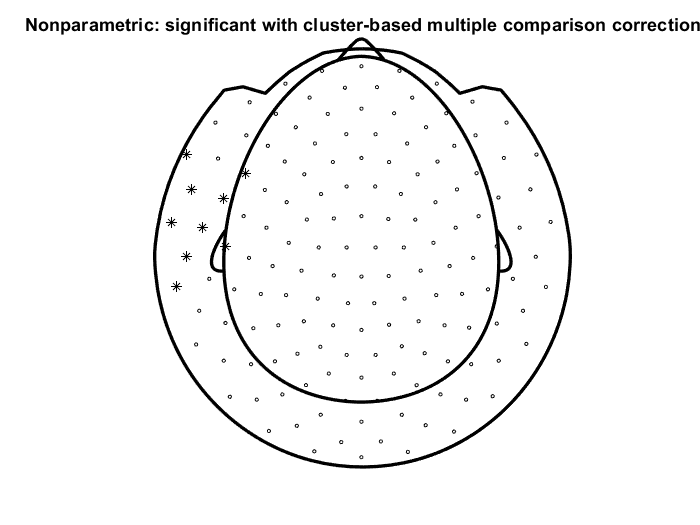Figure 1: Pipeline of statistical testing. All analysis steps in the gray boxes have been done already.

## Reading-in preprocessed and time-locked data in planar gradient format, and grand averaged data

We now describe how we can statistically test the difference between the event-related averages for fully incongruent (FIC) and the fully congruent (FC) sentence endings. For this analysis we use planar gradient data. For convenience we will not do the reading-in and preprocessing steps on all subjects. Instead we begin by loading the timelock structures containing the event-related averages (of the planar gradient data) of all ten subjects. You can download the subject averages. We will also make use of the function ft_timelockgrandaverage to calculate the grand average (average across subjects) and plot it to visually inspect the data

``````  load ERF_orig;    % averages for each individual subject, for each condition
``````

ERF_orig contains allsubjFIC and allsubjFC, each storing the event-related averages for the fully incongruent, and the fully congruent sentence endings, respectively.

The format for these variables, are a prime example of how you should organise your data to be suitable for timelock, freq and source statistics. Specifically, each variable is a cell-array of structures, with each subject’s averaged stored in one cell. To create this data structure two steps are required. First, the single-subject averages were calculated individually for each subject using the function ft_timelockanalysis. Second, using a for-loop we have combined the data from each subject, within each condition, into one variable (allsubj_FIC/allsubj_FC). We suggest that you adopt this procedure as well.

On a technical note, it is preferred to represent the multi-subject data as a cell-array of structures, rather than a so-called struct-array. The reason for this is that the cell-array representation allows for easy expansion into a MATLAB function that allows for a variable number of input arguments (which is how the timelock, freq and source statistics functions have been designed).

## Parametric statistics

### A single comparison (t-test)

#### Plotting the grand-average and the subject-averages

It is good practice to visually inspect your data at the different stages of your analysis. Below we show a couple ways of plotting data: the grand average (averaged across all subjects) for all channels and one specific channel, as well as plotting the individual average for multiple subjects next to each other.

To begin with we will compute the grand average data using ft_timelockgrandaverage

``````% load individual subject data

% calculate grand average for each condition
cfg = [];
cfg.channel   = 'all';
cfg.latency   = 'all';
cfg.parameter = 'avg';
grandavgFIC  = ft_timelockgrandaverage(cfg, allsubjFIC{:});
grandavgFC   = ft_timelockgrandaverage(cfg, allsubjFC{:});
% "{:}" means to use data from all elements of the variable
``````

Now plot all channels with ft_multiplotER, and channel MLT12 with ft_singleplotER using the grand average data.

``````cfg = [];
cfg.showlabels  = 'yes';
cfg.layout      = 'CTF151_helmet.mat';
figure; ft_multiplotER(cfg, grandavgFIC, grandavgFC)

cfg = [];
cfg.channel = 'MLT12';
figure; ft_singleplotER(cfg, grandavgFIC, grandavgFC)
``````From the grand average plot we can zoom in on our comparison of interest and only plot the ERF of channel MLT12 for all subjects, using the individual subject averages data.

``````time = [0.3 0.7];
% Scaling of the vertical axis for the plots below
ymax = 1.9e-13;
figure;
for isub = 1:10
subplot(3,4,isub)
% use the rectangle to indicate the time range used later
rectangle('Position',[time(1) 0 (time(2)-time(1)) ymax],'FaceColor',[0.7 0.7 0.7]);
hold on;
% plot the lines in front of the rectangle
plot(allsubjFIC{isub}.time,allsubjFIC{isub}.avg(52,:), 'b');
plot(allsubjFC{isub}.time,allsubjFC{isub}.avg(52,:), 'r');
title(strcat('subject ',num2str(isub)))
ylim([0 1.9e-13])
xlim([-1 2])
end
subplot(3,4,11);
text(0.5,0.5,'FIC','color','b') ;text(0.5,0.3,'FC','color','r')
axis off
``````From the individual plots and grand average plots above, it seems that between 300ms and 700ms there is a difference between the two conditions in channel MLT12 (channel 52).

We can also plot the differences between conditions, for each subject, in a different manner to further highlight the difference between conditions, using the code below:

``````chan = 52;
time = [0.3 0.7];

% find the time points for the effect of interest in the grand average data
timesel_FIC = find(grandavgFIC.time >= time(1) & grandavgFIC.time <= time(2));
timesel_FC  = find(grandavgFC.time >= time(1) & grandavgFC.time <= time(2));

% select the individual subject data from the time points and calculate the mean
for isub = 1:10
valuesFIC(isub) = mean(allsubjFIC{isub}.avg(chan,timesel_FIC));
valuesFC(isub)  = mean(allsubjFC{isub}.avg(chan,timesel_FC));
end

% plot to see the effect in each subject
M = [valuesFIC(:) valuesFC(:)];
figure; plot(M', 'o-'); xlim([0.5 2.5])
legend({'subj1', 'subj2', 'subj3', 'subj4', 'subj5', 'subj6', ...
'subj7', 'subj8', 'subj9', 'subj10'}, 'location', 'EastOutside');
``````We are starting here with a single-channel analysis here for purely didactical reasons, i.e. start with a simple test without multiple comparisons, and then build up the complexity by adding multiple time points and channels.

In practice you should not guide your statistical analysis by a visual inspection of the data; you should state your hypothesis up-front and avoid data dredging or p-hacking.

#### T-test with MATLAB function

You can do a dependent samples t-test with the MATLAB ttest function (in the Statistics toolbox) where you average over this time window for each condition, and compare the average between conditions. From the output, we look at the output variable ‘stats’ and see that the effect on the selected time and channel is significant with a t-value of -4.9999 and a p-value of 0.00073905.

``````% dependent samples ttest
FICminFC = valuesFIC - valuesFC;
[h,p,ci,stats] = ttest(FICminFC, 0, 0.05) % H0: mean = 0, alpha 0.05
``````

#### T-test with FieldTrip function

You can do the same thing in FieldTrip (which does not require the statistics toolbox) using the ft_timelockstatistics function. This gives you the same p-value of 0.00073905.

``````% define the parameters for the statistical comparison
cfg = [];
cfg.channel     = 'MLT12';
cfg.latency     = [0.3 0.7];
cfg.avgovertime = 'yes';
cfg.parameter   = 'avg';
cfg.method      = 'analytic';
cfg.statistic   = 'ft_statfun_depsamplesT';
cfg.alpha       = 0.05;
cfg.correctm    = 'no';

Nsub = 10;
cfg.design(1,1:2*Nsub)  = [ones(1,Nsub) 2*ones(1,Nsub)];
cfg.design(2,1:2*Nsub)  = [1:Nsub 1:Nsub];
cfg.ivar                = 1; % the 1st row in cfg.design contains the independent variable
cfg.uvar                = 2; % the 2nd row in cfg.design contains the subject number

stat = ft_timelockstatistics(cfg, allsubjFIC{:}, allsubjFC{:});   % don't forget the {:}!
``````

From the code above you can see that the statistical comparison is between conditions (FIC and FC), and that in order to do this you must provide the individual time-locked dataset, from each subject, into the statistics function.

#### Exercise 1

Look at the temporal evolution of the effect by changing cfg.latency and cfg.avgovertime in ft_timelockstatistics. You can plot the t-value versus time, the probability versus time and the statistical mask versus time. Note that the output of the ft_timelockstatistics function closely resembles the output of the ft_timelockanalysis function.

### Multiple comparisons

In the previous paragraph we picked a channel and time window by hand after eyeballing the effect. If you would like to test the significance of the effect in all channels you could make a loop over channels.

``````%loop over channels
time = [0.3 0.7];
timesel_FIC = find(grandavgFIC.time >= time(1) & grandavgFIC.time <= time(2));
timesel_FC  = find(grandavgFC.time >= time(1) & grandavgFC.time <= time(2));
clear h p

FICminFC = zeros(1,10);

for iChan = 1:151
for isub = 1:10
FICminFC(isub) = ...
mean(allsubjFIC{isub}.avg(iChan,timesel_FIC)) - ...
mean(allsubjFC{isub}.avg(iChan,timesel_FC));
end

[h(iChan), p(iChan)] = ttest(FICminFC, 0, 0.05 ); % test each channel separately
end

% plot uncorrected "significant" channels
cfg = [];
cfg.style     = 'blank';
cfg.layout    = 'CTF151_helmet.mat';
cfg.highlight = 'on';
cfg.highlightchannel = find(h);
cfg.comment   = 'no';
figure; ft_topoplotER(cfg, grandavgFIC)
title('significant without multiple comparison correction')
``````However, since now you are performing 151 individual tests, you can no longer control the false alarm rate. Given the null-hypothesis and an alpha of 5%, you have a 5% chance of making a false alarm and incorrectly concluding that the null-hypothesis should be rejected. As this false alarm rate applies to each test that you perform, the chance of making a false alarm for 151 tests in parallel is much larger than the desired 5%. This is the multiple comparison problem.

To ensure that the false alarm rate is controlled at the desired alpha level over all channels, you should do a correction for multiple comparisons. The best known correction is the Bonferroni correction, which controls the false alarm rate for multiple independent tests. To do this, divide your initial alpha level (i.e. the desired upper boundary for the false alarm rate) by the number of tests that you perform. In this case with 151 channels the alpha level would become 0.05/151 which is 0.00033113. With this alpha value the effect is no longer significant on any channel. In some cases, as in this one, a Bonferroni correction is then too conservative because the effect on one MEG channel is highly correlated with neighbouring channels (in space, and also in time). In sum, even though the Bonferroni correction achieves the desired control of the type-I error (false alarm rate), the type-II error (chance of not rejecting H0 when in fact it should be rejected) is much larger than desired.

Below you can see the means by which to implement a Bonferroni correction. However, there are other options on FieldTrip to control for multiple comparisons which are less conservative, and hence more sensitive.

#### Bonferroni correction with MATLAB function

``````% with Bonferroni correction for multiple comparisons
FICminFC = zeros(1,10);

for iChan = 1:151
for isub = 1:10
FICminFC(isub) = ...
mean(allsubjFIC{isub}.avg(iChan,timesel_FIC)) - ...
mean(allsubjFC{isub}.avg(iChan,timesel_FC));
end

[h(iChan), p(iChan)] = ttest(FICminFC, 0, 0.05/151); % test each channel separately
end
``````

#### Bonferroni correction with FieldTrip function

``````cfg = [];
cfg.channel     = 'MEG'; %now all channels
cfg.latency     = [0.3 0.7];
cfg.avgovertime = 'yes';
cfg.parameter   = 'avg';
cfg.method      = 'analytic';
cfg.statistic   = 'ft_statfun_depsamplesT';
cfg.alpha       = 0.05;
cfg.correctm    = 'bonferroni';

Nsub = 10;
cfg.design(1,1:2*Nsub)  = [ones(1,Nsub) 2*ones(1,Nsub)];
cfg.design(2,1:2*Nsub)  = [1:Nsub 1:Nsub];
cfg.ivar                = 1; % the 1st row in cfg.design contains the independent variable
cfg.uvar                = 2; % the 2nd row in cfg.design contains the subject number

stat = ft_timelockstatistics(cfg, allsubjFIC{:}, allsubjFC{:});
``````

FieldTrip also has other methods implemented for performing a multiple comparison correction, such as FDR. See the statistics_analytic function for the options to cfg.correctm when you want to do a parametric test.

## Non-parametric statistics

### Permutation test based on t statistics

Instead of using the analytic t-distribution to calculate the appropriate p-value for your effect, you can use a nonparametric randomization test to obtain the p-value.

This is implemented in FieldTrip in the function ft_statistics_montecarlo which is called by ft_timelockstatistics when you set cfg.method = ‘montecarlo’. A Monte-Carlo estimate of the significance probabilities and/or critical values is calculated based on randomising or permuting your data many times between the conditions.

``````cfg = [];
cfg.channel     = 'MEG';
cfg.latency     = [0.3 0.7];
cfg.avgovertime = 'yes';
cfg.parameter   = 'avg';
cfg.method      = 'montecarlo';
cfg.statistic   = 'ft_statfun_depsamplesT';
cfg.alpha       = 0.05;
cfg.correctm    = 'no';
cfg.correcttail = 'prob';
cfg.numrandomization = 'all';  % there are 10 subjects, so 2^10=1024 possible permutations

Nsub = 10;
cfg.design(1,1:2*Nsub)  = [ones(1,Nsub) 2*ones(1,Nsub)];
cfg.design(2,1:2*Nsub)  = [1:Nsub 1:Nsub];
cfg.ivar                = 1; % the 1st row in cfg.design contains the independent variable
cfg.uvar                = 2; % the 2nd row in cfg.design contains the subject number

stat = ft_timelockstatistics(cfg, allsubjFIC{:}, allsubjFC{:})

% make the plot
cfg = [];
cfg.style     = 'blank';
cfg.layout    = 'CTF151_helmet.mat';
cfg.highlight = 'on';
cfg.comment   = 'no';
figure; ft_topoplotER(cfg, grandavgFIC)
title('Nonparametric: significant without multiple comparison correction')
``````

With the method (cfg.method) of statistical test set as a permutation-based test (montecarlo) the following channels contribute to the significant difference between the conditions (p<0.05) between 0.3 and 0.7 s.Compare this plot with the earlier one using parametric statistics with the uncorrected p-values.

Also in the non-parametric approach for testing of statistical significance different corrections for multiple comparisons such as Bonferroni, fdr, and others are implemented. See the options for cfg.correctm in statistics_montecarlo.

### Permutation test based on cluster statistics

FieldTrip also implements a special way to correct for multiple comparisons, which makes use of the feature in the data that the effects at neighbouring timepoints and channels are highly correlated. For more details see the cluster permutation tutorials for ERFs and time frequency data and the publication by Maris and Oostenveld (2007).

This method requires you to define neighbouring channels. FieldTrip has a function that can do that for you called ft_prepare_neighbours. The following example will construct a neighbourhood structure and show which channels are defined as neighbours:

``````cfg = [];
cfg.method      = 'template';                         % try 'distance' as well
cfg.template    = 'ctf151_neighb.mat';                % specify type of template
cfg.layout      = 'CTF151_helmet.mat';                % specify layout of channels
cfg.feedback    = 'yes';                              % show a neighbour plot
neighbours      = ft_prepare_neighbours(cfg, grandavgFIC); % define neighbouring channels

% note that the layout and template fields have to be entered because at the earlier stage
% when ft_timelockgrandaverage is called the field 'grad' is removed. It is this field that
% holds information about the (layout of the) channels.

cfg = [];
cfg.channel     = 'MEG';
cfg.neighbours  = neighbours; % defined as above
cfg.latency     = [0.3 0.7];
cfg.avgovertime = 'yes';
cfg.parameter   = 'avg';
cfg.method      = 'montecarlo';
cfg.statistic   = 'ft_statfun_depsamplesT';
cfg.alpha       = 0.05;
cfg.correctm    = 'cluster';
cfg.correcttail = 'prob';
cfg.numrandomization = 'all';  % there are 10 subjects, so 2^10=1024 possible permutations

Nsub = 10;
cfg.design(1,1:2*Nsub)  = [ones(1,Nsub) 2*ones(1,Nsub)];
cfg.design(2,1:2*Nsub)  = [1:Nsub 1:Nsub];
cfg.ivar                = 1; % the 1st row in cfg.design contains the independent variable
cfg.uvar                = 2; % the 2nd row in cfg.design contains the subject number

stat = ft_timelockstatistics(cfg, allsubjFIC{:}, allsubjFC{:});

% make a plot
cfg = [];
cfg.style     = 'blank';
cfg.layout    = 'CTF151_helmet.mat';
cfg.highlight = 'on';
cfg.comment   = 'no';
figure; ft_topoplotER(cfg, grandavgFIC)
title('Nonparametric: significant with cluster-based multiple comparison correction')
``````

With the cluster-based permutation method for multiple comparisons the following channels contribute to the significant difference between the conditions (p<0.05) between 0.3 and 0.5 s.So far we predefined a time window over which the effect was averaged, and tested the difference of that between conditions. You can also chose to not average over the predefine time window, and instead cluster simultaneously over neighbouring channels and neighbouring time points within your time window of interest . From the example below, we now find a channel-time cluster is found from 0.33 s until 0.52 s in which p < 0.05.

``````cfg = [];
cfg.channel     = 'MEG';
cfg.neighbours  = neighbours; % defined as above
cfg.latency     = [-0.25 1];
cfg.avgovertime = 'no';
cfg.parameter   = 'avg';
cfg.method      = 'montecarlo';
cfg.statistic   = 'ft_statfun_depsamplesT';
cfg.alpha       = 0.05;
cfg.correctm    = 'cluster';
cfg.correcttail = 'prob';
cfg.numrandomization = 'all';  % there are 10 subjects, so 2^10=1024 possible permutations
cfg.minnbchan        = 2;      % minimal number of neighbouring channels

Nsub = 10;
cfg.design(1,1:2*Nsub)  = [ones(1,Nsub) 2*ones(1,Nsub)];
cfg.design(2,1:2*Nsub)  = [1:Nsub 1:Nsub];
cfg.ivar                = 1; % the 1st row in cfg.design contains the independent variable
cfg.uvar                = 2; % the 2nd row in cfg.design contains the subject number

stat = ft_timelockstatistics(cfg, allsubjFIC{:}, allsubjFC{:});
``````

ft_clusterplot can be used to plot the effect.

``````% make a plot
cfg = [];
cfg.highlightsymbolseries = ['*','*','.','.','.'];
cfg.layout = 'CTF151_helmet.mat';
cfg.contournum = 0;
cfg.markersymbol = '.';
cfg.alpha = 0.05;
cfg.parameter='stat';
cfg.zlim = [-5 5];
ft_clusterplot(cfg, stat);
``````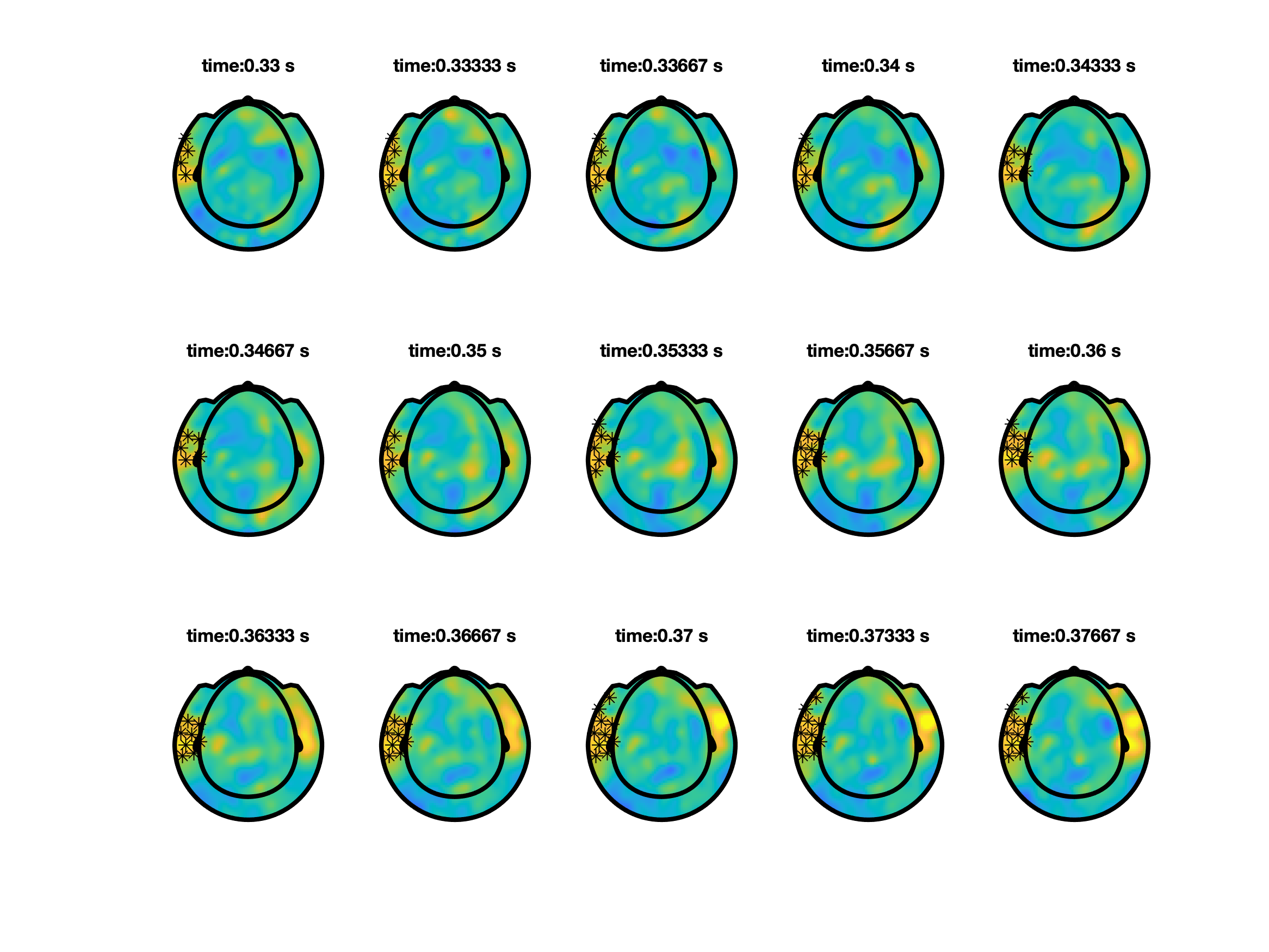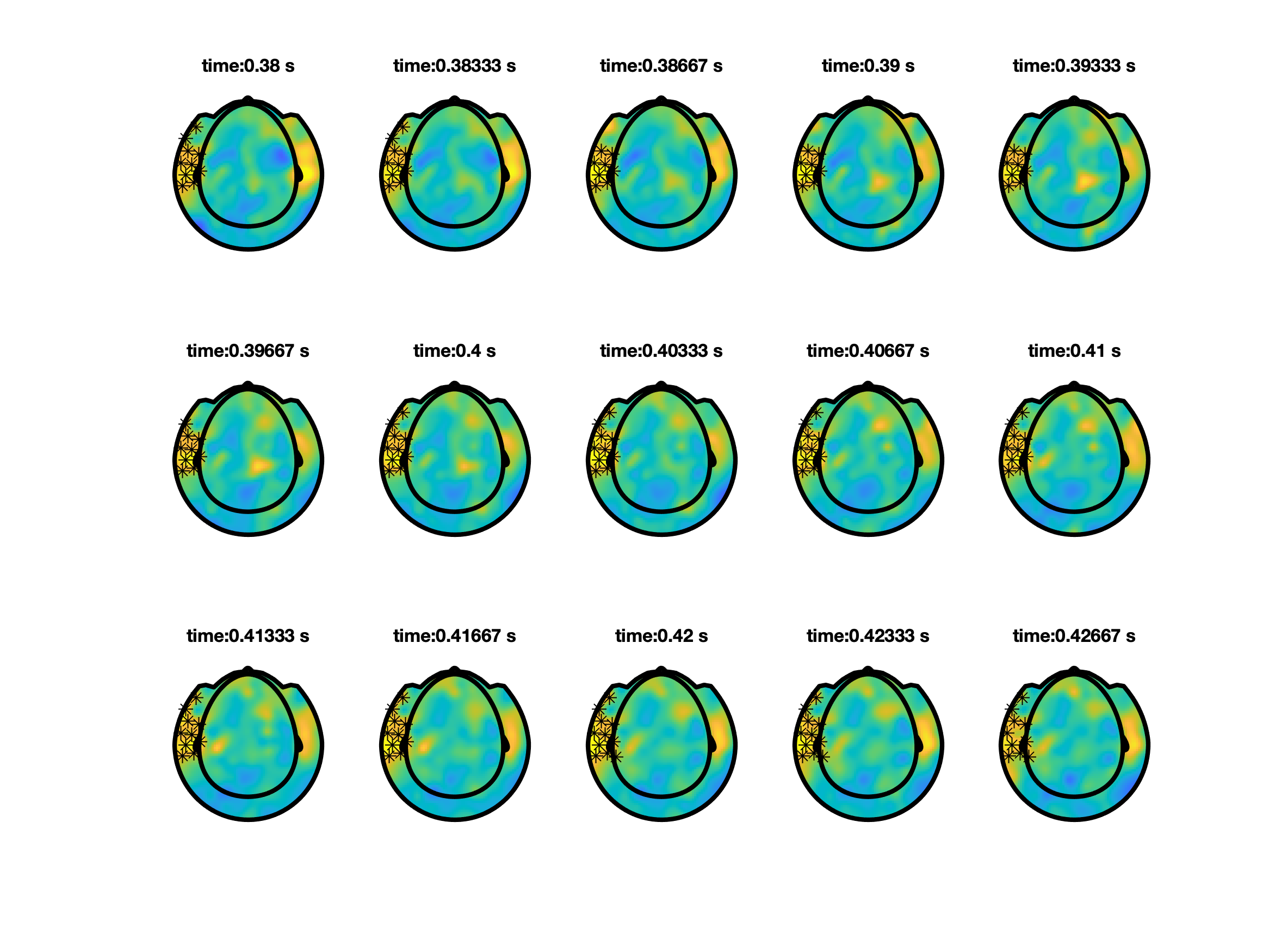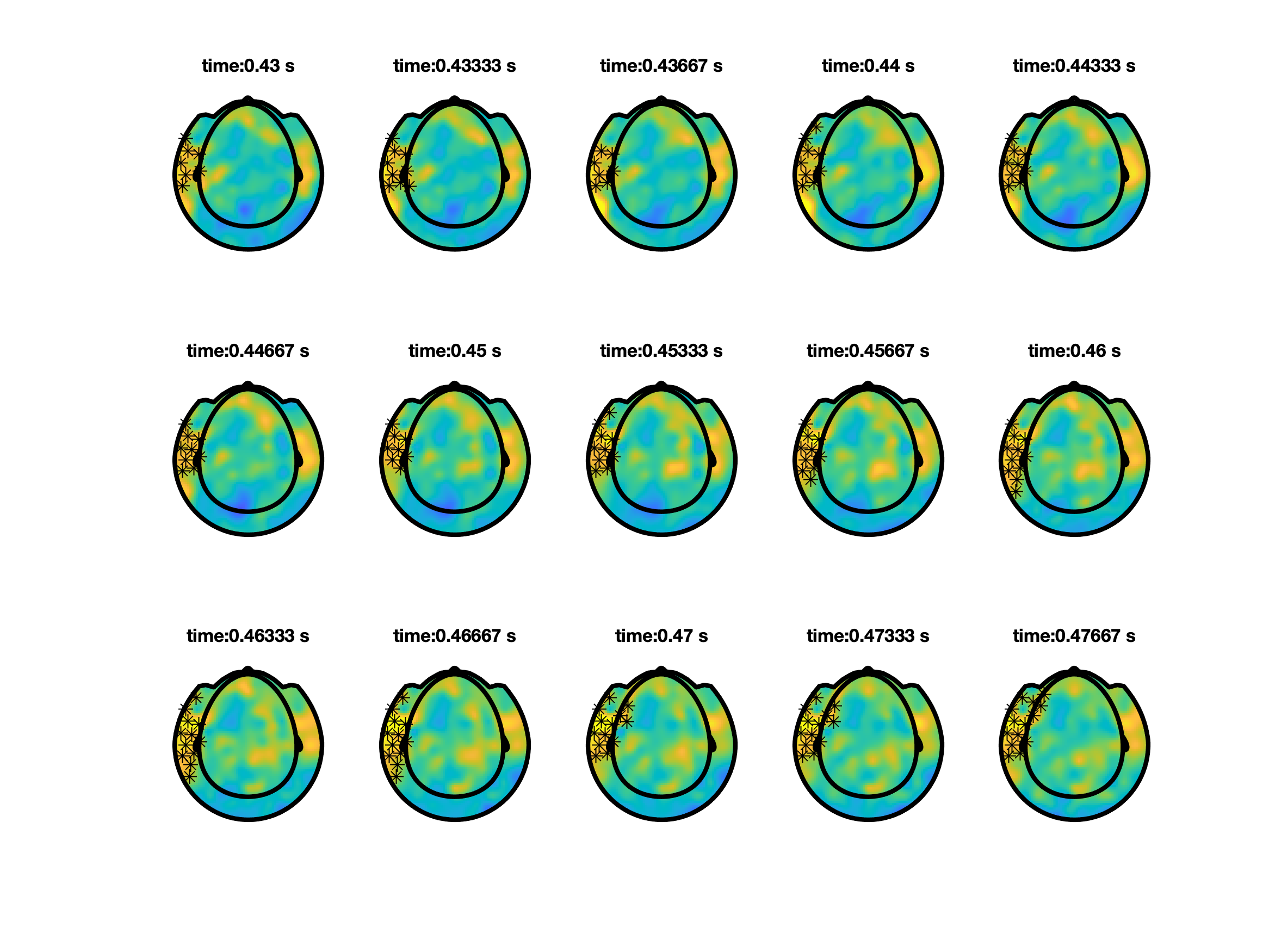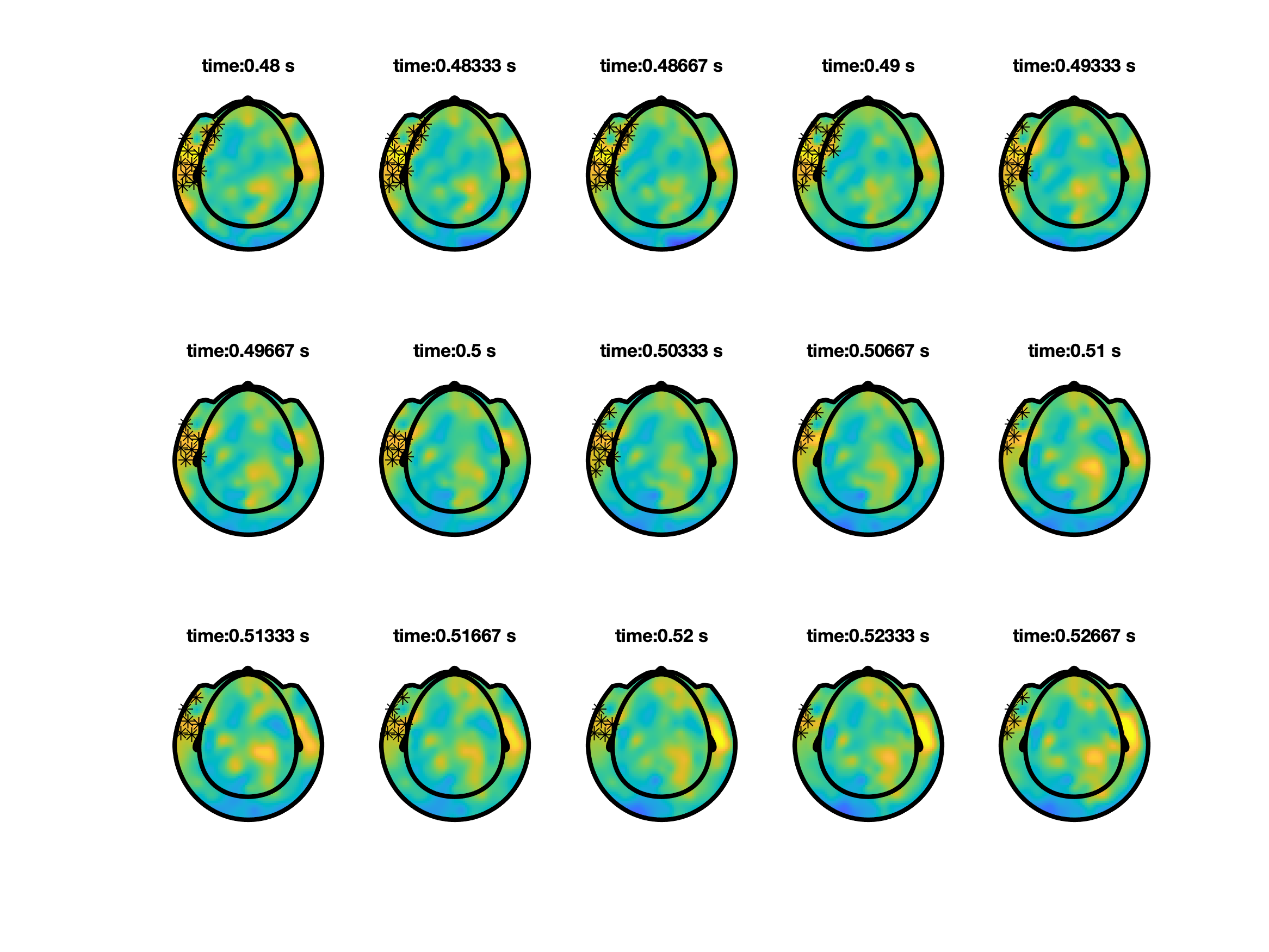To properly write up your results in a manuscript, you should check the guidelines on how NOT to interpret results from a cluster-based permutation test.

## Summary and suggested further reading

This tutorial showed you how to perform parametric and non-parametric statistics in FieldTrip, as well as the the equivalent t-test and Bonferroni correction with MATLAB functions. Furthermore, it demonstrated how to plot the channels that contribute to a significant difference.

After this gentle introduction in statistics with FieldTrip, you can continue with the Cluster-based permutation tests on event-related fields and the Cluster-based permutation tests on time-frequency data tutorials. These give a more detailed description of non-parametric statistical testing with a cluster-based approach.

If you would like to read more about statistical analysis, you can look at the following FAQs:

If you would like to read about neighbourhood selection, you can read the following FAQs:

And you can look also at the following example scripts: